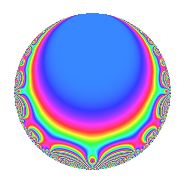# Properties

 Label 6027.2.a.pLevel 6027 Weight 2 Character orbit 6027.a Self dual Yes Analytic conductor 48.126 Analytic rank 1 Dimension 3 CM No Inner twists 1

# Related objects

## Newspace parameters

 Level: $$N$$ = $$6027 = 3 \cdot 7^{2} \cdot 41$$ Weight: $$k$$ = $$2$$ Character orbit: $$[\chi]$$ = 6027.a (trivial)

## Newform invariants

 Self dual: Yes Analytic conductor: $$48.1258372982$$ Analytic rank: $$1$$ Dimension: $$3$$ Coefficient field: 3.3.1620.1 Coefficient ring: $$\Z[a_1, \ldots, a_{5}]$$ Coefficient ring index: $$1$$ Fricke sign: $$1$$ Sato-Tate group: $\mathrm{SU}(2)$

## $q$-expansion

Coefficients of the $$q$$-expansion are expressed in terms of a basis $$1,\beta_1,\beta_2$$ for the coefficient ring described below. We also show the integral $$q$$-expansion of the trace form.

 $$f(q)$$ $$=$$ $$q$$ $$- q^{3}$$ $$-2 q^{4}$$ $$-\beta_{1} q^{5}$$ $$+ q^{9}$$ $$+O(q^{10})$$ $$q$$ $$- q^{3}$$ $$-2 q^{4}$$ $$-\beta_{1} q^{5}$$ $$+ q^{9}$$ $$+ ( -\beta_{1} + \beta_{2} ) q^{11}$$ $$+ 2 q^{12}$$ $$+ ( -1 - \beta_{1} - \beta_{2} ) q^{13}$$ $$+ \beta_{1} q^{15}$$ $$+ 4 q^{16}$$ $$-2 q^{17}$$ $$+ ( -3 - \beta_{1} ) q^{19}$$ $$+ 2 \beta_{1} q^{20}$$ $$+ ( 2 + \beta_{1} ) q^{23}$$ $$+ ( 3 + 2 \beta_{1} + \beta_{2} ) q^{25}$$ $$- q^{27}$$ $$+ 2 \beta_{1} q^{29}$$ $$+ ( 1 + 2 \beta_{1} - \beta_{2} ) q^{31}$$ $$+ ( \beta_{1} - \beta_{2} ) q^{33}$$ $$-2 q^{36}$$ $$- q^{37}$$ $$+ ( 1 + \beta_{1} + \beta_{2} ) q^{39}$$ $$- q^{41}$$ $$+ ( -7 - \beta_{2} ) q^{43}$$ $$+ ( 2 \beta_{1} - 2 \beta_{2} ) q^{44}$$ $$-\beta_{1} q^{45}$$ $$+ ( -\beta_{1} - 3 \beta_{2} ) q^{47}$$ $$-4 q^{48}$$ $$+ 2 q^{51}$$ $$+ ( 2 + 2 \beta_{1} + 2 \beta_{2} ) q^{52}$$ $$+ ( -2 - 2 \beta_{1} - 2 \beta_{2} ) q^{53}$$ $$+ ( 10 + 2 \beta_{1} + 3 \beta_{2} ) q^{55}$$ $$+ ( 3 + \beta_{1} ) q^{57}$$ $$+ ( 6 + 2 \beta_{1} + 2 \beta_{2} ) q^{59}$$ $$-2 \beta_{1} q^{60}$$ $$+ ( 2 + 2 \beta_{1} + 3 \beta_{2} ) q^{61}$$ $$-8 q^{64}$$ $$+ ( 6 + 3 \beta_{1} - \beta_{2} ) q^{65}$$ $$+ ( 1 - \beta_{1} - \beta_{2} ) q^{67}$$ $$+ 4 q^{68}$$ $$+ ( -2 - \beta_{1} ) q^{69}$$ $$+ ( 2 \beta_{1} + 2 \beta_{2} ) q^{71}$$ $$+ ( -7 - \beta_{2} ) q^{73}$$ $$+ ( -3 - 2 \beta_{1} - \beta_{2} ) q^{75}$$ $$+ ( 6 + 2 \beta_{1} ) q^{76}$$ $$+ ( 7 - \beta_{1} + 2 \beta_{2} ) q^{79}$$ $$-4 \beta_{1} q^{80}$$ $$+ q^{81}$$ $$+ ( -2 - \beta_{1} - 4 \beta_{2} ) q^{83}$$ $$+ 2 \beta_{1} q^{85}$$ $$-2 \beta_{1} q^{87}$$ $$+ ( 4 + \beta_{1} + 3 \beta_{2} ) q^{89}$$ $$+ ( -4 - 2 \beta_{1} ) q^{92}$$ $$+ ( -1 - 2 \beta_{1} + \beta_{2} ) q^{93}$$ $$+ ( 8 + 5 \beta_{1} + \beta_{2} ) q^{95}$$ $$+ ( 6 - 4 \beta_{1} ) q^{97}$$ $$+ ( -\beta_{1} + \beta_{2} ) q^{99}$$ $$+O(q^{100})$$ $$\operatorname{Tr}(f)(q)$$ $$=$$ $$3q$$ $$\mathstrut -\mathstrut 3q^{3}$$ $$\mathstrut -\mathstrut 6q^{4}$$ $$\mathstrut +\mathstrut 3q^{9}$$ $$\mathstrut +\mathstrut O(q^{10})$$ $$3q$$ $$\mathstrut -\mathstrut 3q^{3}$$ $$\mathstrut -\mathstrut 6q^{4}$$ $$\mathstrut +\mathstrut 3q^{9}$$ $$\mathstrut +\mathstrut 6q^{12}$$ $$\mathstrut -\mathstrut 3q^{13}$$ $$\mathstrut +\mathstrut 12q^{16}$$ $$\mathstrut -\mathstrut 6q^{17}$$ $$\mathstrut -\mathstrut 9q^{19}$$ $$\mathstrut +\mathstrut 6q^{23}$$ $$\mathstrut +\mathstrut 9q^{25}$$ $$\mathstrut -\mathstrut 3q^{27}$$ $$\mathstrut +\mathstrut 3q^{31}$$ $$\mathstrut -\mathstrut 6q^{36}$$ $$\mathstrut -\mathstrut 3q^{37}$$ $$\mathstrut +\mathstrut 3q^{39}$$ $$\mathstrut -\mathstrut 3q^{41}$$ $$\mathstrut -\mathstrut 21q^{43}$$ $$\mathstrut -\mathstrut 12q^{48}$$ $$\mathstrut +\mathstrut 6q^{51}$$ $$\mathstrut +\mathstrut 6q^{52}$$ $$\mathstrut -\mathstrut 6q^{53}$$ $$\mathstrut +\mathstrut 30q^{55}$$ $$\mathstrut +\mathstrut 9q^{57}$$ $$\mathstrut +\mathstrut 18q^{59}$$ $$\mathstrut +\mathstrut 6q^{61}$$ $$\mathstrut -\mathstrut 24q^{64}$$ $$\mathstrut +\mathstrut 18q^{65}$$ $$\mathstrut +\mathstrut 3q^{67}$$ $$\mathstrut +\mathstrut 12q^{68}$$ $$\mathstrut -\mathstrut 6q^{69}$$ $$\mathstrut -\mathstrut 21q^{73}$$ $$\mathstrut -\mathstrut 9q^{75}$$ $$\mathstrut +\mathstrut 18q^{76}$$ $$\mathstrut +\mathstrut 21q^{79}$$ $$\mathstrut +\mathstrut 3q^{81}$$ $$\mathstrut -\mathstrut 6q^{83}$$ $$\mathstrut +\mathstrut 12q^{89}$$ $$\mathstrut -\mathstrut 12q^{92}$$ $$\mathstrut -\mathstrut 3q^{93}$$ $$\mathstrut +\mathstrut 24q^{95}$$ $$\mathstrut +\mathstrut 18q^{97}$$ $$\mathstrut +\mathstrut O(q^{100})$$

Basis of coefficient ring in terms of a root $$\nu$$ of $$x^{3}\mathstrut -\mathstrut$$ $$12$$ $$x\mathstrut -\mathstrut$$ $$14$$:

 $$\beta_{0}$$ $$=$$ $$1$$ $$\beta_{1}$$ $$=$$ $$\nu$$ $$\beta_{2}$$ $$=$$ $$\nu^{2} - 2 \nu - 8$$
 $$1$$ $$=$$ $$\beta_0$$ $$\nu$$ $$=$$ $$\beta_{1}$$ $$\nu^{2}$$ $$=$$ $$\beta_{2}\mathstrut +\mathstrut$$ $$2$$ $$\beta_{1}\mathstrut +\mathstrut$$ $$8$$

## Embeddings

For each embedding $$\iota_m$$ of the coefficient field, the values $$\iota_m(a_n)$$ are shown below.

For more information on an embedded modular form you can click on its label.

Label $$\iota_m(\nu)$$ $$a_{2}$$ $$a_{3}$$ $$a_{4}$$ $$a_{5}$$ $$a_{6}$$ $$a_{7}$$ $$a_{8}$$ $$a_{9}$$ $$a_{10}$$
1.1
 3.94338 −1.39091 −2.55247
0 −1.00000 −2.00000 −3.94338 0 0 0 1.00000 0
1.2 0 −1.00000 −2.00000 1.39091 0 0 0 1.00000 0
1.3 0 −1.00000 −2.00000 2.55247 0 0 0 1.00000 0
 $$n$$: e.g. 2-40 or 990-1000 Significant digits: Format: Complex embeddings Normalized embeddings Satake parameters Satake angles

## Inner twists

This newform does not admit any (nontrivial) inner twists.

## Atkin-Lehner signs

$$p$$ Sign
$$3$$ $$1$$
$$7$$ $$1$$
$$41$$ $$1$$

## Hecke kernels

This newform can be constructed as the intersection of the kernels of the following linear operators acting on $$S_{2}^{\mathrm{new}}(\Gamma_0(6027))$$:

 $$T_{2}$$ $$T_{5}^{3}$$ $$\mathstrut -\mathstrut 12 T_{5}$$ $$\mathstrut +\mathstrut 14$$ $$T_{13}^{3}$$ $$\mathstrut +\mathstrut 3 T_{13}^{2}$$ $$\mathstrut -\mathstrut 15 T_{13}$$ $$\mathstrut -\mathstrut 35$$